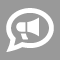#Blogs

## Math Word Problem Blogs

I should have done this in March. This year it took me 1 week (after work hours) to complete all the puzzles.  The last puzzle is NOT friendly to full time  shift employees (hint). There are only 2 right answers; one answer must be done during scheduled work hours and the other answer must be done after any normal person goes to bed at night (or early morning).   It's still not too late to get onto http://www.pidaychallenge.com and work through 24 puzzles to get onto the Genius board. You may work at the puzzles at your own pace.  Stats stayed consistent to previous years; the total set of puzzles are still hard enough that only approximately 10% successfully work all problems.  Maybe, there is an endurance factor :-)  There were logic, probability, algebra, geometry, and physics problems/puzzles/questions. Enjoy the challenge!

Michael spent the following amounts of time building a bookcase: drawing up the plans: 2 hours cutting wood: 1 ½ hours assembling the bookcase: 2 hours Sanding and sealing: 3 ½ hours   What is the ratio of time spent cutting the wood to total time spent on the project? 1:9 1:6 1:5 3:7   Solution: We need to find two pieces of information in order to solve this problem: Time spent cutting the wood Total time spent on the project We find that it took 1 ½ hours to cut the wood from looking above at the information provided in the question. Next, find the time the entire project took by adding up all the times. We get 9 hours to complete the whole project. Now we solve our problem by doing the following steps: Our ratio is: time spent cutting wood : total time spent of the project We plug in the numbers to get: 1 ½:9 We multiply each term of the ratio by... read more

In math or science we come across terms such as inverse proportion and direct proportion. When two variables are directly proportional an increase in one variable causes an increase in the other variable. When two variables are inversely proportional an increase in one variable causes a decrease in the other variable.    Inverse Proportion: To illustrate inverse proportionality, I will use a common physics problem. Two golf balls are thrown down from a tall building at the same time and one ball has twice the velocity of the other ball. Which ball hits the ground first assuming only velocity is different?  We already know that velocity is approximately equal to distance / time. Let the velocity of the slower ball be v. Assuming only the velocity of the two balls is different, we can say approximately v = d / t. We can eliminate wind force, atmospheric force, and force of gravity since both balls will be affected equally. If you increase v,... read more

Often times experienced mathematicians tend to get comfortable with certain problem-solving strategies. For example, in a problem one might use a system of equations to solve a problem rather than employing a simpler more easy way to solve it. Though using system of equations are great, knowing how to solve problems using different approaches is important, not just for oneself, but for their students.   Take for example the following problem: A farmer has both pigs and chickens on his farm. There are 78 feet and 27 heads. How many pigs and how many chickens are there?   Solution 1: (Using Algebra System of Equations) 4p+2c=78 (pigs have 4 feet and chickens have 2 feet with 78 feet in total) p+c=27 (27 heads mean that the number of chicken and pigs total 27) Then by algebra p=27-c. Therefore by substitution, 4(27-c)+2c=78. 108-2c=78. 2c=30. c=15. Since, c=15, p+c=p+15=27. p=12. Therefore, the farmer has 15 chickens and 12 pigs... read more